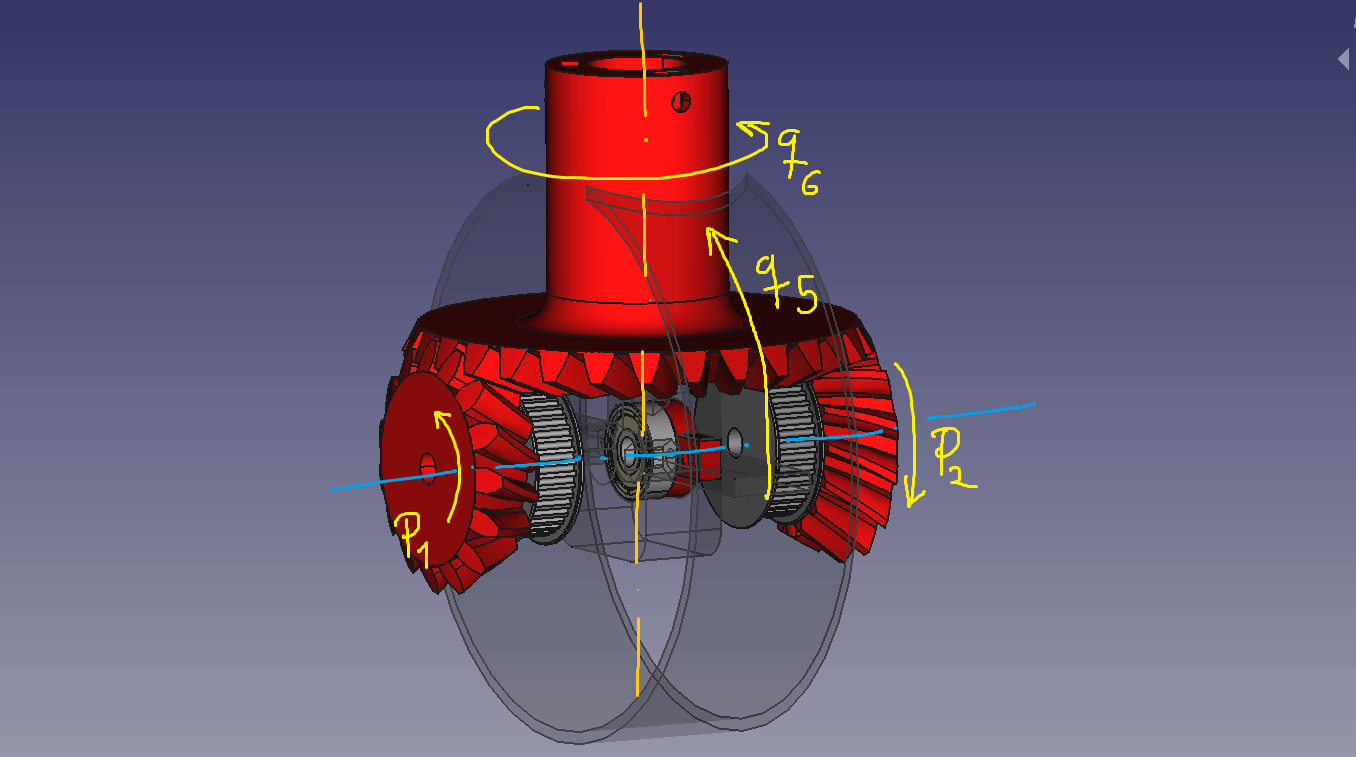# Reply To: Programacion de articulaciones con mas de un motor

#1899

I think the solution is as follows if we take into account the following sign convention for rotations:p1 represents the angle rotated by pinion 1.
p2 represents the angle rotated by pinion 2.
q5 and q6 refer to the angles of joints 5 and 6 respectively.

I think these equations are correct:
q5 = (p1-p2)/2
q6 = r*(p1+p2)/2
r = pinion teeths / crown wheel teeths

I have obtained these equations by an empirical analysis of the problem and not by analyzing in depth the theory of the mechanism, so I could be wrong.

I hope it helps!